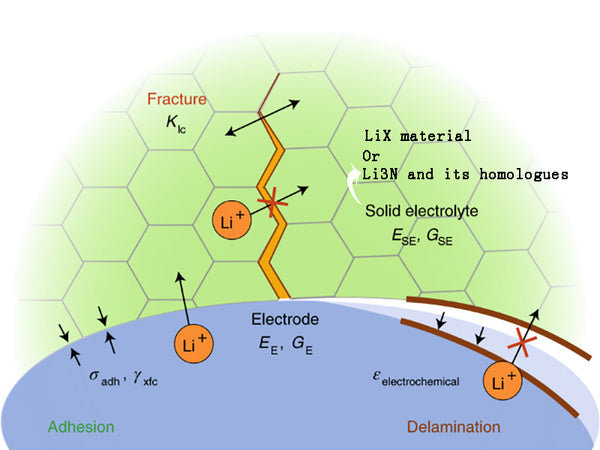• 1. LiX material
• 2. Li3N and its homologues
•All LiX (X=F, Cl, Br and I) materials have a NaCl type crystal structure. Except for LiI, they are almost perfect ionic crystals, which are insulators at room temperature. Due to the strong polarity of I-, the chemical bond of LiI is covalent to a certain extent, and the conductivity of Li+ at 30°C can reach 5.5×10-7S/cm. Although the conductivity of LiI is higher than that of other LiX, it is still about 6 orders of magnitude lower than that of RbAg4I5. However, it should be pointed out that LiI film was the first solid electrolyte material used in Li/I2 (composite) batteries in 1972. The LiI film is very thin, and the internal resistance of the entire battery is very small. The battery made can be used in small current power supply occasions, such as pacemakers.

To improve the conductivity of LiX solid electrolyte, doping with isomeric cations such as CaI2 and trying to synthesize double salt LiAlCl4.

The LiI-Al2O3 dispersive solid electrolyte is prepared by mixing anhydrous LiI and Al2O3 powder with high specific surface area in an ammonia atmosphere with a water content of less than 15μg/g, and then sintering the mixture at 500°C for 17h and cooling. Then re-grind and press into a cake (green) to obtain a solid electrolyte sample.

The ionic conductivity in the LiI-Al2O3 system has nothing to do with the generated defects. Dispersing Al2O3 in LiI can increase the conductivity by 2 orders of magnitude. It is very common to use dispersed dielectric materials such as Al2O3 to increase the ionic conductivity of other halogen-based cation conductors such as CuCl. The smaller the particle radius of the dielectric material, the more obvious this effect. Under normal circumstances, when the additive reaches a certain concentration, the conductivity will have a maximum value.

This phenomenon can be caused by the space charge layer composed of V'Li (lithium ion vacancies) and LiI (lithium ion interstitial) generated at the interface between the host material LiI (or CuCl) and the dielectric particles. Contribute to explain. Suppose a small particle of Al2O3 with a radius of r1 is placed in the center of a CuCl (or LiI) particle with a radius of r2 [Figure 1(a)]. At this time, a space charge layer with a thickness of 1m is induced around the Al2O3 particles [Figure 1(b)], λ is called the Debye length, which can be expressed as:

λ≈(8πNe²/εkT)e(﹣E/kT)1/2=[(8πe²/εkT)n∞]1/2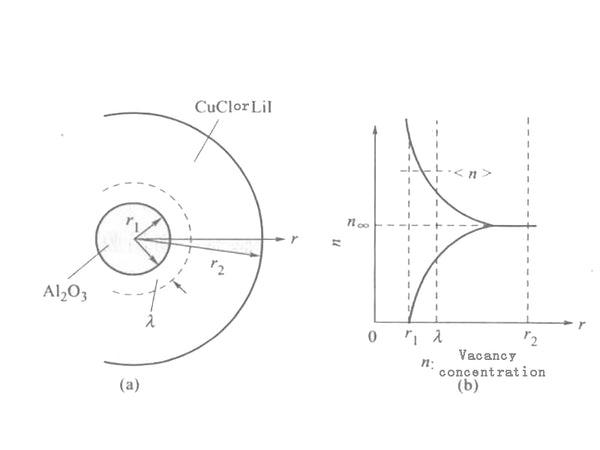Figure 1 CuCl (or Lil)-Al2O3, simulation of the formation of vacancies in solid electrolyte

In the formula, N is the total number of cations; ℇ is the dielectric constant; E is the formation energy of defects; n is the defect concentration in the area away from the interface. Assuming that the defect concentration in the range of r1~(r1+λ) is , then the conductivity σ due to the conduction of the grain boundary layer can be expressed as:

σb≈eμ[(4πr²1λ)/4π/3(r32﹣r31)]∝(1/r1)×(ν/1﹣ν)

In the formula, v is the volume ratio of Al2O3, where r2≫r1 is assumed. The above formula is applicable to both CuCl-Al2O3 and LiI-Al2O3 systems.

The composite metal halide Li2MX4 having an inverse spinel structure is an example of a halogen-type Li+ conductor. The reported relationship between the conductivity σ and 1/T of the compound together with the phase transition observed by differential thermal analysis (DTA) and high temperature ray diffraction techniques is shown in Figure 2. The inflection point in Figure 2 corresponds to the phase transition, but in the case of the cubic spinel structure, this inflection point is related to the order-disorder transition of the Li+ position between the six-coordinate positions of the tetragonal phase and the mesophase.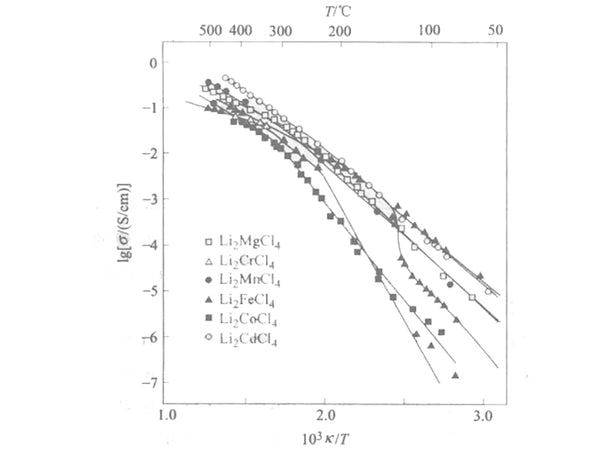Figure 2 Li2MCl4 system (M is Mg, Cr, Mn, Fe, Co and Cd) the electrical conductivity between σ and 1/T curve

The Li2S-P2S5 glass with a room temperature lithium ion conductivity exceeding 10-4S/cm was prepared by mechanical ball milling, and then the Li2S-P2S5 glass was heat treated above the crystallization temperature to obtain Li2S-P2S5 glass ceramics with higher room temperature lithium ion conductivity Its electrical conductivity reaches 10-3S/cm. Experiments have found that Li2S-SiS2 fusion-quenched glass containing a small amount of oxides has higher room temperature lithium ion conductivity than materials using pure sulfides. When stainless steel is used as a blocking electrode, the DC conductivity of the glass ceramics of both components decreases with time due to polarization, so adding a small amount of P2O5 can reduce the electronic conductivity of the electrolyte. The lithium ion migration number in such a glass-ceramic electrolyte is close to 1; a reported thio-LISICON solid electrolyte: Li3.25Ge0.25P0.75S4 has a room temperature conductivity of 2.2×10-3S/cm, and has a high electrical conductivity. It is chemically stable, does not react with metallic lithium, and does not undergo phase change up to 500°C. These characteristics make this electrolyte membrane particularly suitable as an electrolyte material for all-solid-state lithium-ion batteries. In a very narrow composition range [in which Li2S accounts for 66.7% (mole fraction)], the amorphous solid electrolyte Li2S-P2S3 was obtained by high-energy ball milling. The maximum ionic conductivity of the electrolyte 66.7Li2S·33.3P2S3 (mole fraction, %) at room temperature reaches 1.1×10-4S/cm. Cyclic voltammetry measurements show that the electrochemical window of the sample is about 5V.

2. Li3N and its homologues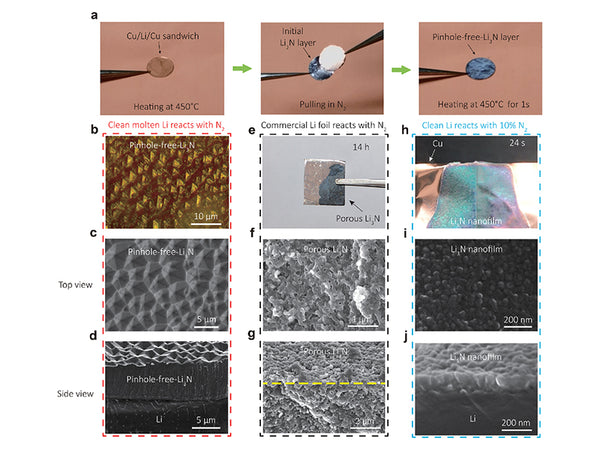The ionic compound lithium nitride (Li3N) can be prepared by the direct reaction of metallic lithium with nitrogen. In the hexagonal system, the crystal is composed of an infinitely long chain of six-sided double pyramids, with N3-located in the center of the pyramid. The bond length of N-Li(1) [Li(1) at the top of the pyramid] is 0.194nm, while N-Li(2)[Li(2) at the six corners of the hexagonal plane] is 0.211nm. Therefore, it has a layered structure as a whole, in which Li(2)2N and Li(1) are stacked alternately. Li+ conducts electricity mainly along the plane composed of Li(1). This has been confirmed by the measurement of single crystal Li3N: at 25℃, the conductivity along the direction parallel to the c-axis is 1.2×10-3S/cm, and the conductivity along the direction perpendicular to the c-axis is 1 ×10-5S/cm. In addition, the conductivity of the polycrystalline sample has been reported to be 7×10-4S/cm.

Since the conductivity of ordinary Li3N is generally 104 times higher than that of LiI or 102 times higher than that of LiI-Al2O3, it is a solid electrolyte material with many applications. But one of the main disadvantages of this material is that the decomposition voltage is too low, only 0.445V. At least in theory, it is impossible to use it as an electrolyte to build batteries with an electromotive force higher than 0.445V. In order to solve this problem, various Li3N-based compound systems have been studied.

Figure 3 lists the lithium ion conductivity of some compounds. Among them, the ternary system Li3N-LiI-LiOH (molar ratio 1:2:0.77) obtained by sintering the mixture in a nitrogen atmosphere at 550°C for 3 hours is the most successful. The electrical conductivity of this material is as high as 0.95×10-3S/cm, which is almost the same as that of Li3N, while its decomposition voltage is as high as 1.6~1.8V. In addition, its electron migration number is lower than 10-5. Such high conductivity may be attributed to the arrangement of the anions (N3- and OH-) of the material's bcc structure in the crystal.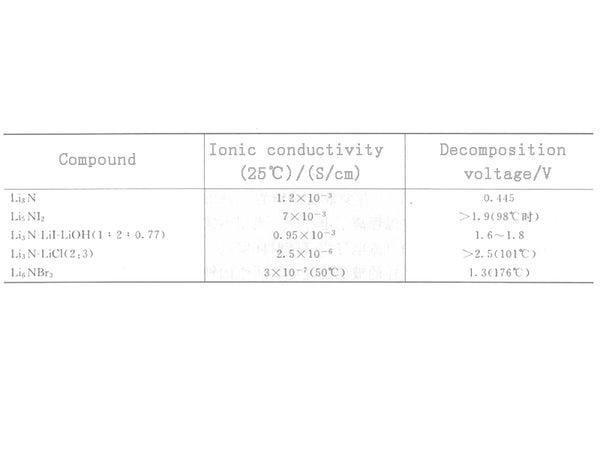Figure 3 Comparison of the conductivity of Li3N and its derivatives